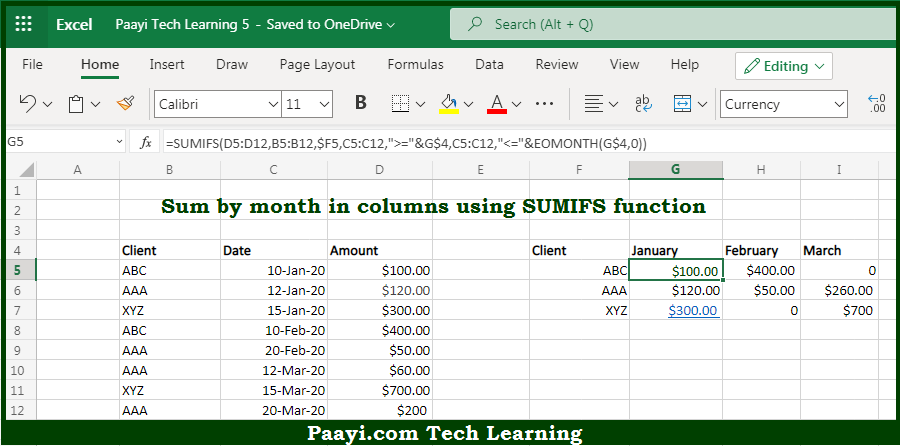# Learn How to SUM By Month in Columns in Microsoft Excel

Written by | 0 Comments | 555 Views

In this article, you will learn how to COUNT various things in Microsoft Excel using a single/combination(s) of functions. You will also know how to SUM By Month in Columns and see the generic formula.

SUM By Month in Columns in Microsoft Excel

The main purpose of this formula is to sum by month in columns. Here we will learn how to SUM by month in the given columns in Microsoft Excel. That implies, with the help of a formula based on the SUMIFS and EOMONTH function you can able to sum by month in columns. So, with the help of this formula, you can able to SUM by month in the given columns in Microsoft Excel.

General Formula to SUM By Month in Columns

=SUMIFS(amount,client,\$F5,date,">="&G\$4,date,"<="&EOMONTH(G\$4,0))

The Explanation for the SUM By Month in ColumnsSo we know that with the help of the given formula above you can able to sum by month in columns. Here we will learn how to SUM by month in the given columns in Microsoft Excel. As we know that the inside the SUMIFS function is designed to sum values in a range based on one or more criteria. In this case, we need three criteria, that is - Client name = client in column F, then Date >= first of the month, and finally the Date <= end of the month. While coming back to the SUMIFS function, the first range/criteria pair is used to check the client name. So, with the help of this formula, you can able to SUM by month in the given columns in Microsoft Excel.